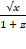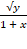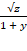Chapter 16.5, Problem 5E

Chapter
Section
Textbook Problem

Find (a) the curl and (b) the divergence of the vector field.5. x, y, z) =i +j +k

(a)

To determine

To find: The curl F.

Explanation

Given data:

F(x,y,z)=x1+zi+y1+xj+z1+yk .

Formula used:

Consider the standard equation of an curl F for F=Pi+Qj+Rk .

curlF=|ijkxyzPQR| (1)

Substitute x1+z for P , y1+x for Q and z1+y for R in equation (1),

curlF=|ijkxyzx1+zy1+xz1+y|={[y(z1+y)z(y1+x)]i[x(z1+y)z(x1+z)]j+[x(y1+x)y(x1+z)]k}={

(b)

To determine

To find: The divergence of the vector field.

Still sussing out bartleby?

Check out a sample textbook solution.

See a sample solution

The Solution to Your Study Problems

Bartleby provides explanations to thousands of textbook problems written by our experts, many with advanced degrees!

Get Started

11. Is the graph of concave up or concave down at x = 2?

Mathematical Applications for the Management, Life, and Social Sciences

Proof Prove die second case of Theorem 4.6.

Calculus: Early Transcendental Functions (MindTap Course List)

The range of is: (−∞,∞) [0, ∞) (0, ∞) [1, ∞]

Study Guide for Stewart's Multivariable Calculus, 8th

Let f(x)={2x2forx13forx1. Which limit does not exist? a) limx3f(x) b) limx1+f(x) c) limx1f(x) d) limx1f(x)

Study Guide for Stewart's Single Variable Calculus: Early Transcendentals, 8th